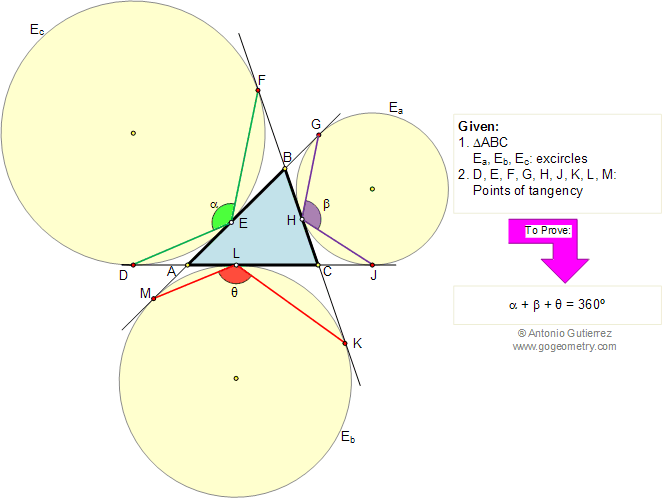Problem 208. Triangle, Excircles, Angles, 360 degrees
 In the figure below, given a triangle ABC, Ea, Eb, and Ec are the three excircles, and the points D, E, F, G, H, J, K, L, and M are the points of tangency. Prove that the sum of the angles DEF, GHJ, and KLM is 360 degrees. View or post a solution.Home | Geometry | Search | Problems | 201-210 | View or post a solution | Email